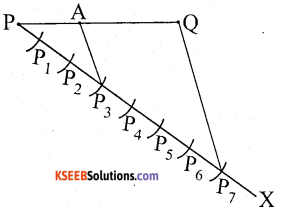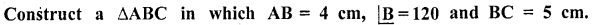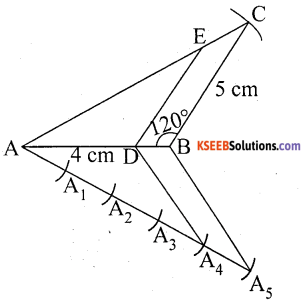# KSEEB Solutions for Class 10 Maths Chapter 6 Constructions Additional Questions

Students can Download Class 10 Maths Chapter 6 Constructions Additional Questions, Notes Pdf, KSEEB Solutions for Class 10 Maths helps you to revise the complete Karnataka State Board Syllabus and score more marks in your examinations.

## Karnataka State Syllabus Class 10 Maths Chapter 6 Constructions Additional Questions

Question 1.
Construct a triangle similar to a given triangle ABC with its sides equal to 5/3 of the corresponding sides of triangle ABC.BDE is the corresponding similar triangle to ABC.

Question 2.
Draw a line segment PQ 7 cm and divide it in the ratio 3 : 4
Ratio 3 : 4 = 3 + 4 = 7The point A divides PQ
∴ PA : AQ = 3 : 4

Question 3.
Draw a circle of radius 4 cm and chord of length is 6 cm. Construct tangents at the ends of chord.∴ PQ and RS are two required tangents.

Question 4.Construct another ∆ ADE similar to ∆ ABC. Such that AD = $$\frac{4}{5}$$AB∴ ADE is the required similar triangle

Question 5.
Draw a circle of radius 3.5 cm. Take two points P and Q on one of its extended diameter each at a distance of 7 cm from its centre. Draw tangents to the circle from these two points P and Q.∴ PA and PB are two required tangents from an external point P.
∴ QC and QD are two required from an external point Q.

error: Content is protected !!